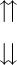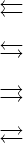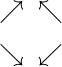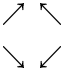# How to denote arrow symbol in LaTeX?

Arrow symbols play an important role in our lives. And these arrow symbols are divided into different parts according to direction, structure, and shape. In this tutorial, I will try to represent all types of arrow symbols with the help of latex.

Notice the table below, where the fundamental arrow symbols are denoted by latex.

 Arrow type Command / Symbol Up arrow \uparrow   ↑ Down arrow \downarrow  ↓ Right arrow \rightarrow  → Left arrow \leftarrow  ← N-W arrow \nwarrow   ↖ N-E arrow \nearrow   ↗ S-E arrow \searrow    ↘ S-W arrow \swarrow   ↙ Up double arrow \Uparrow ⇑ Left double arrow \Leftarrow ⇐ Right double arrow \Rightarrow ⇒ Down double arrow \Downarrow ⇓

In this tutorial, we will cover each of the fundamental arrows, as well as various other arrow-type symbols that are used in the document.

## Up arrow in LaTeX

LaTeX has the default \uparrow and \Uparrow commands to represent the up arrow symbol. However, although the spellings of the two commands are the same, the first letter of  \uparrow command is in the lower case and the first letter of \Uparrow command is in the upper case.

Thus, the two commands represent different values. Because latex is case-sensitive.

\documentclass{article}
\begin{document}
$\uparrow$
$\Uparrow$
\end{document}

Output :

↑
⇑

If you want to make the arrow symbol bigger, you need to use the \big command before the \uparrow command.

\documentclass{article}
\begin{document}
$\uparrow$
$\big\uparrow$
\end{document}

Output :

↑
↑

Using the \uparrow command indirect text mode will show you a syntax error. For this, you need to take the help of textcomp package, which has a  defined special \textuparrow command.

\documentclass{article}
\usepackage{textcomp}
\begin{document}
% You can directly use arrow(↑) symbol in text mode
Up arrow symbol(\textuparrow)
\end{document}

Output :

Up arrow symbol (↑)

## Down arrow in LaTeX

Putting down instead of up in the \uparrow command will turn each up arrow symbol into a down arrow. For example

\documentclass{article}
\usepackage{textcomp}
\begin{document}
Down arrow symbol : $\downarrow$
Double down arrow symbol : $\Downarrow$
Big down arrow symbol : $\big\downarrow$
% down arrow in text mode
Down arrow symbol : \textdownarrow
\end{document}

Output :

Down arrow symbol : ↓
Double down arrow symbol : ⇓
Big down arrow symbol : ↓ Down arrow symbol : ↓

## Up down arrow in LaTeX

In the case of double up-down arrows, there will be a total of four combinations. For example ⇈, ⇅, ⇵, and ⮇.

\documentclass{article}
\usepackage{amssymb}
\begin{document}
$\upuparrows$
$\downdownarrows$
\end{document}

Output :However, up-down and down-up arrows are not in the amssymb package. For this, you need to use the mathabx package.

\documentclass{article}
\usepackage{mathabx}
\begin{document}
$\upuparrows$
$\updownarrows$
$\downdownarrows$
$\downuparrows$
\end{document}

Output :

⇈
⇅
⇵
⮇


Depending on the shape of the symbol, it is better to use mathabx package instead of amssymb package.

## Right Arrow in LaTeX

In the case of right arrow, the main command is \rightarrow.

\documentclass{article}
\usepackage{textcomp}
\begin{document}
Right arrow symbol : $\rightarrow$
Double right arrow symbol : $\Rightarrow$
Big right arrow symbol : $\longrightarrow$
% right arrow in text mode
Right arrow symbol : \textrightarrow
\end{document}

Output :

Right arrow symbol : →
Right double arrow symbol : ⇒
Right long arrow symbol : ⟶
Right text arrow symbol : →
Left arrow in LaTeX

## Left arrow in LaTeX

And in case of left arrow, the default latex command is \leftarrow.

\documentclass{article}
\usepackage{textcomp}
\begin{document}
Left arrow symbol : $\leftarrow$
Double left arrow symbol : $\Leftarrow$
Big left arrow symbol : $\longleftarrow$
% left arrow in text mode
Left arrow symbol : \textleftarrow
\end{document}

Output :

Left arrow symbol : ←
Left double arrow symbol : ⇐
Left long arrow symbol : ⟵
Left text arrow symbol : ←


## Double Left-right arrow in LaTeX

Similarly, in the case of a double left-right arrow, there will be four combinations. For example ⮄, ⮀, ⮂, and ⮆.

\documentclass{article}
\usepackage{amssymb}
\begin{document}
$\leftleftarrows$
$\leftrightarrows$
$\rightrightarrows$
$\rightleftarrows$
\end{document}

Output :The mathabx package contains the same commands to represent the above symbols. For example

\documentclass{article}
\usepackage{amssymb}
\begin{document}
$\leftleftarrows$
$\leftrightarrows$
$\rightrightarrows$
$\rightleftarrows$
\end{document}

Output :

⮄
⮀
⮆
⮂


## Diagonal arrow in LaTeX

The default diagonal arrow symbols have \nearrow, \nwarrow, \searrow, and \swarrow commands.

\documentclass{article}
\begin{document}
$\nearrow \;\; \nwarrow$
$\searrow \;\; \swarrow$
\end{document}

Output :However, according to the shape of the symbol, using the mathabx package is best practice and the same command is present for the diagonal arrow in this package.

\documentclass{article}
\usepackage{mathabx}
\begin{document}
$\nearrow \;\; \nwarrow$
$\searrow \;\; \swarrow$
\end{document}

Output :#### Md Jidan Mondal

LaTeX expert with over 10 years of experience in document preparation and typesetting. Specializes in creating professional documents, reports, and presentations using LaTeX.